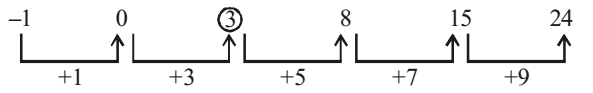## Number Series

#### Number Series

Direction: What will come in the place of question mark (?) in the following number.

1. –1, 0, ?, 8, 15, 24
1. 4
2. 3
3. 2
4. 1
5. None of these

1. The number pattern is described in the below image.It is clear that we are getting the next terms on adding + 1 , + 3 , + 5 , + 7 , + 9 in previous term respectively.
First term = - 1
Second term = First term + 1
Third term = Second term + 3
Similarly we can calculate the all others terms

##### Correct Option: B

The number pattern is described in the below image.
As per figure we can see the number series is in specific pattern which will help us to find the next number.
It is clear that we are getting the next terms on adding + 1 , + 3 , + 5 , + 7 , + 9 in previous term respectively.
First term = - 1
Second term = First term + 1
Second term = -1 + 1 = 0
Third term = Second term + 3
Third term = 0 + 3 = ( ? )
∴ ( ? ) = 3
Similarly we can calculate the all others terms
Fourth term = 8
Fifth term= 151. 1, 2, 6, 24, ? ,720
1. 3
2. 5
3. 120
4. 8
5. None of these

1. As per given series , we can see that
First term = 1
Second term = First term × 2 = 2
Third term = Second term × 3 = 6
Similarly we can calculate the all others terms

##### Correct Option: C

As per given series , we can see that
First term = 1
Second term = First term × 2
Second term = 1 × 2 = 2
Similarly we can find the all others terms
Third term = Second term × 3
Third term = 2 × 3 = 6
Fourth term = Third term × 4
Fourth term = 6 × 4 = 24
Fifth term = Fourth term × 5
Fifth term = 24 × 5 = ?
∴ ? = 120
Sixth term = Fifth term × 6
Sixth term = 120 × 6 = 720

1. 251, (12), 107, 381, (?) , 125
1. 14
2. 24
3. 11
4. 16
5. None of these

1. The given series is in the below pattern,we can see that
Square root of ( First term - Third term ) = Second term
⇒ Square root of ( 251 – 107 ) = Square root of 144 = 12 = Second term
Square root of ( Fourth term - sixth term ) = Fifth term
⇒ 381 – 125 = Square root of 256 = 16 = Fifth term

##### Correct Option: D

The given series is in the below pattern,we can see that
Square root of ( First term - Third term ) = Second term
⇒ Square root of ( 251 – 107 ) = Square root of 144 = 12 = Second term
Square root of ( Fourth term - sixth term ) = Fifth term
⇒ 381 – 125 = Square root of 256 = 16 = Fifth term
Fifth term ( ? ) = 16
Hence, 16 is the missing number in the given question.

1. 3120, ?, 122, 23, 4
1. 488
2. 621
3. 610
4. 732
5. None of these

1. As per above given series , we can see that
Descending order of given series -
Next term = ( 5 x previous term ) + odd number ( which starts 3 )
Here , 5th term = 4
4th term = 5 × 5th term + 3 = 5 × 4 + 3 = 23
3rd term= 5 × 4th term + 7 = 5 × 23 + 7 = 122
Similarly , we can calculate all others terms also.

##### Correct Option: B

As per above given series , we can see that
Descending order of given series -
Next term = ( 5 x previous term ) + odd number ( which starts 3 )
Here , 5th term = 4
4th term = 5 × 5th term + 3
4th term = 5 × 4 + 3 = 23
3rd term= 5 × 4th term + 7
3rd term= 5 × 23 + 7 = 122
Similarly , we can find all others terms also.
2nd term = 5 × 3rd term + 11
2nd term = 5 × 122 + 11 = ?
? = 621
1st term = 5 × 2nd term + 15
1st term = 5 × 621 + 15 =3120